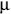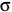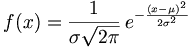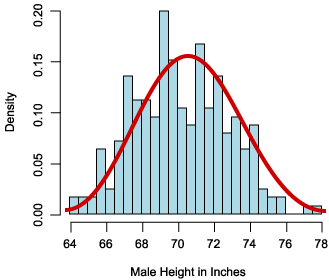Search

Useful Lists

Partners

Online Manuals

Apply probability models to Web data using PHP
By Paul Meagher - 2004-04-14 Page:  1 2 3 4 5 6 7 8 9 10 11

## Model fitting

In the model-fitting stage, your goal is to replace the observed probability distribution with a better understood theoretical probability distribution. This substitution enables you to more easily make probability statements about your random variable (variables such as "What is the probability of meeting someone seven feet tall?"; "What is the probability of getting 10 orders this week?"; or "What is the probability of getting a visitor to the Web site in the next 10 minutes?").

When you look at the observed probability distribution for male height, it appears to have a symmetrical bell shape that is reminiscent of the plot for a normally distributed random variable. This observation suggests that you should do your model fitting by comparing the observed distribution of heights to the distribution of heights predicted using a normal probability distribution.

If you can establish that the difference between the observed and predicted height distributions is small, then you can use the normal distribution to assign probability values for various statements about male height (or the probability that a hard drive will fail within a certain period of time, or the probability of having X number of motor vehicle accidents this week, and so on).

Before you can compare the observed distribution with the distribution predicted using the normal distribution, you must first compute the mean and standard deviation of the observed distribution. This is because the normal probability distribution function which generates the best-fitting normal distribution accepts a mean and standard deviation as adjustment parameters.

In the normal distribution function in Figure 3, you can see that the mean deviation (Mu,) and the standard deviation (sigma,) appear to be fixed parameters in the formula:

Figure 3. Formula for normal distribution functionThe formula returns the probability density associated with each height value.

The mean and standard deviation parameters are used to tweak the location and shape of the normal distribution. The observed estimates are used as the most likely candidates for the mean and standard deviation of the best-fitting normal distribution curve. Graphically speaking, Figure 4 demonstrates that supplying the parameters should cause the theoretical normal distribution (red line) to overlay the observed distribution so that goodness of fit can be visually assessed.

Figure 4. Using deviation parameters to visually assess fitThe red line is the plot of the probability densities for each height value between 64 and 78 using a mean of 70.31 and a standard deviation of 2.61 as the formula parameters.

Use the following PHP script to generate the normal density values as depicted by the red line. You might also find it instructive to compare the textbook formula (Figure 3) with the PHP implementation of this formula. (Note in particular that the fixed parameters, the mean and standard deviation, can be represented as instance variables which are set via a constructor, while the x values are supplied as the variable argument to the normal probability density function, or PDF.)

Listing 1. PHP script for generating normal density values
 `````` mean = \$mu; \$this->stdev = \$sigma; } function PDF(\$x) { \$denominator = sqrt(2 * pi()) * \$this->stdev; \$numerator = exp(-(\$x - \$this->mean) * (\$x - \$this->mean) / ( 2 * \$this->stdev)); \$density = \$numerator / \$denominator; return \$density; } } \$norm = new Normal(70.31, 2.61); echo \$norm->PDF(70); // answer is 0.15 ?> ``````

To assess how good the fit is between the observed probability distribution and a normal distribution, you could generate expected frequencies for each height interval based on what would be expected from a normally distributed random variable with a mean of 70.31 and a standard deviation 2.61. You then could compare the difference between the observed and expected frequencies for each height interval.

If you summed the square of each such difference score and divided by the number of difference scores, you could use the size of this value to indicate whether a normal distribution is a good fit for the data. This obtained value is known as the obtained Chi Square value. The Chi Square value can be used to analyze Web polls, stats, and other data streams, as well as to assess goodness of fit between obtained and theoretical distributions. (See Resources for an article on the use of the Chi Square test.)

The Chi Square test is not the only test you can use to establish goodness-of-fit between theoretical and observed distributions. In the case of male height, a better test to apply is the Kolmogorov-Smirnov test or the Anderson-Darling test. The Kolmogorov-Smirnov test is designed to test the hypothesis that a given data set could have been drawn from a given distribution. Unlike the Chi Square test, it is primarily intended for use with continuous distributions and is independent of arbitrary computational choices such as bin width. The Anderson-Darling test (a modification of Kolmogorov-Smirnov) is used to test if a sample of data came from a population with a specific distribution. It gives more weight to the tails than does Kolmogorov-Smirnov. Kolmogorov-Smirnov is distribution-free in the sense that the critical values do not depend on the specific distribution being tested. Anderson-Darling makes use of the specific distribution in calculating critical values, potentially affording the advantage of allowing a more sensitive test and suffering the disadvantage that critical values must be calculated for each distribution.

My reason for mentioning other common goodness-of-fit tests is that you want to use the best test for the job. The Chi Square test has the virtue that it can be used to assess model fit for most distributions, although it may be less sensitive than other goodness-of-fit tests for particular distributions.

Next, look at how to perform goodness of fit using the Chi Square test. I'll apply it to an example for which it is arguably more appropriate than male height (for example, Anderson-Darling normality test might be better) -- determining whether the numbers generated by PHP's `mt_rand` function can be fit to a uniform distribution.

View Apply probability models to Web data using PHP Discussion

Page:  1 2 3 4 5 6 7 8 9 10 11 Next Page: Is mt_rand() really random?